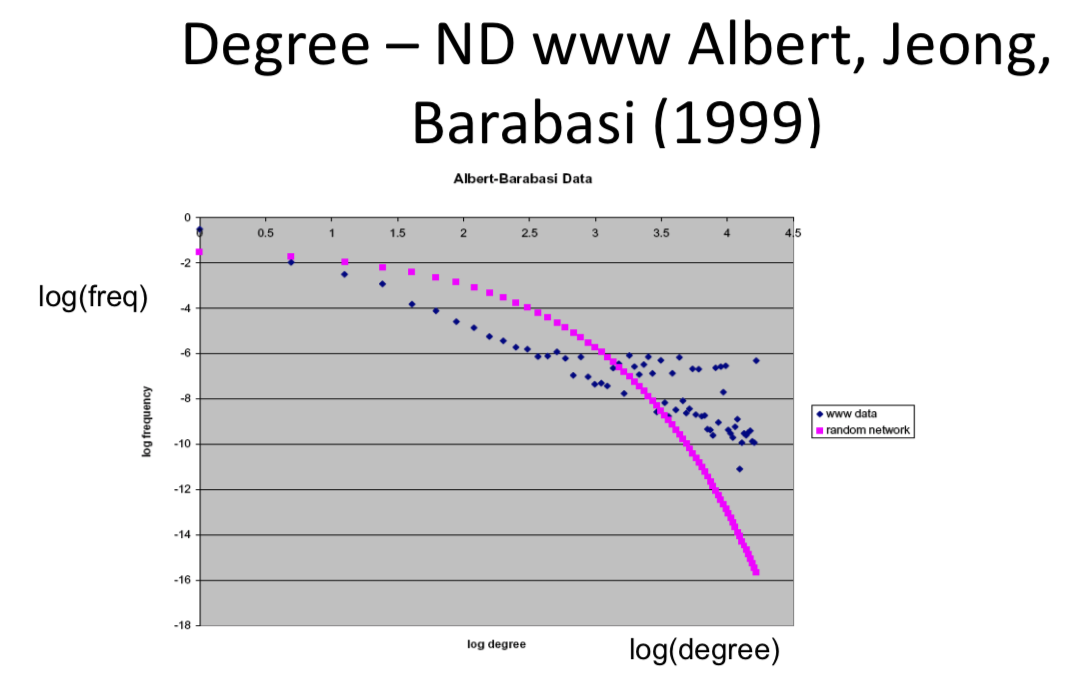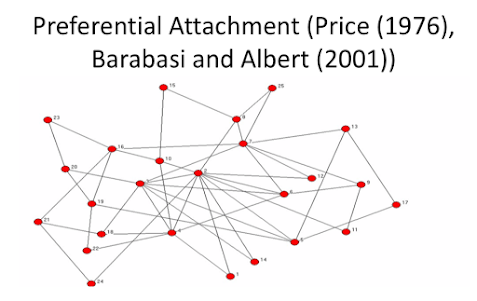# 3.2 Mean Field Approximations¶

Let's have a deeper look at these growing random network models, and in particular we're going to start talking just about Mean Field Approxiamtions, a useful technique for these kinds of models.

## Continuous Time Approximation¶

Continuous time approximation will allow us to just solve a differential equation to figure out what the nodes expected degrees should be over time. Let's go back and think again about simple Erdos-Renyi variation where now each node is born, forms m links at random to the existing ones. But we'll just smooth this out and do a continuous time approximation.

• starting condition => $d_i(i) = m$

• a node i is born so its degree at time i is going to be m, so when its first is born, it forms its m new links.
• new links gained per unit time => $dd_i(t)/dt = m/t$

• then how does this degree change as we change time? What's the differential of the degree of i with respect to time t? Well, this differential is expecting to gain m links over the time period, so there's t existing nodes, m new links being formed, so its chance of getting one of those is m/t. So it's gain per unit of time is going to be propotional to m/t.
• $d_i(t) = m + m log(t/i)$

• It's exactly the same equation as before and then you can just do the same of the calculation where we try and figure out how many nodes have degree less than 35 at some time t.
• More high and low degree nodes than predicted at random

• Citation Networks - too many with 0 citations, too many with high numbers of citations to have citations drawn at random
• "Fat tails" compared to random network
• Related to other settings (wealth, city size, word usage...)## Power Law Explanations¶

Rich get richer - more links you have, the easier it is to get links, so more wealth you get, the easier it is to get more wealth. The bigger your city is, the easier it is to get more population. These kinds of things where you get a multiplicative growth together with new objects being born over time, so new articles, new cities, in this case new nodes. Those things being grown born over time are going to gain proportionally to how large they already are and we'll end up with power loss

## Preferential Attachment (Price(1975), Barabasi and Albert(2001))¶

• Previous models don't have the "fat tals" of degree distributions
• Instead of forming links at random with existing nodes uniformly ar random, the probability that links are going to form is going to be proportional to the number of links that a node already has. This going to be the rich get richer part and that's the preferential attachment I prefer to attach to nodes that already have high numbers of links.